# Shader Toy Tutorial - Glow#

Graphics cards can run programs written in the C-like language OpenGL Shading Language, or GLSL for short. These programs can be easily parallelized and run across the processors of the graphics card GPU.

Shaders take a bit of set-up to write. The ShaderToy website has standardized some of these and made it easier to experiment with writing shaders. The website is at:

## PyCon 2022 Slides#

This tutorial is scheduled to be presented at 2022 PyCon US. Here are the slides for that presentation:

## Step 1: Open a window#

This is simple program that just opens a basic Arcade window. We’ll add a shader in the next step.

Open a window#
  1 2 3 4 5 6 7 8 9 10 11 12 13 14 15 16 import arcade # Derive an application window from Arcade's parent Window class class MyGame(arcade.Window): def __init__(self): # Call the parent constructor super().__init__(width=1920, height=1080) def on_draw(self): # Clear the screen self.clear() if __name__ == "__main__": MyGame() arcade.run() 

This program will load a GLSL program and display it. We’ll write our shader in the next step.

  1 2 3 4 5 6 7 8 9 10 11 12 13 14 15 16 17 18 19 20 21 22 import arcade from arcade.experimental import Shadertoy # Derive an application window from Arcade's parent Window class class MyGame(arcade.Window): def __init__(self): # Call the parent constructor super().__init__(width=1920, height=1080) # Load a file and create a shader from it shader_file_path = "circle_1.glsl" window_size = self.get_size() self.shadertoy = Shadertoy.create_from_file(window_size, shader_file_path) def on_draw(self): # Run the GLSL code self.shadertoy.render() if __name__ == "__main__": MyGame() arcade.run() 

Note

The proper way to read in a file to a string is using a with statement. For clarity/brevity our code isn’t doing that in the presentation. Here’s the proper way to do it:

file_name = "circle_1.glsl"
with open(file_name) as file:


## Step 3: Write a shader#

Next, let’s create a simple first GLSL program. Our program will:

• Normalize the coordinates. Instead of 0 to 1024, we’ll go 0.0 to 1.0. This is standard practice, and allows us to work independently of resolution. Resolution is already stored for us in a standardized variable named iResolution.

• Next, we’ll use a white color as default. Colors are four floating point RGBA values, ranging from 0.0 to 1.0. To start with, we’ll set just RGB and use 1.0 for alpha.

• If we are greater that 0.2 for our coordinate (20% of screen size) we’ll use black instead.

• Set our output color, standardized with the variable name fracColor.

GLSL code for creating a shader.#
  1 2 3 4 5 6 7 8 9 10 11 12 13 14 15 16 17 void mainImage(out vec4 fragColor, in vec2 fragCoord) { // Normalized pixel coordinates (from 0 to 1) vec2 uv = fragCoord/iResolution.xy; // How far is the current pixel from the origin (0, 0) float distance = length(uv); // Are we are 20% of the screen away from the origin? if (distance > 0.2) { // Black fragColor = vec4(0.0, 0.0, 0.0, 1.0); } else { // White fragColor = vec4(1.0, 1.0, 1.0, 1.0); } } 

The output of the program looks like this: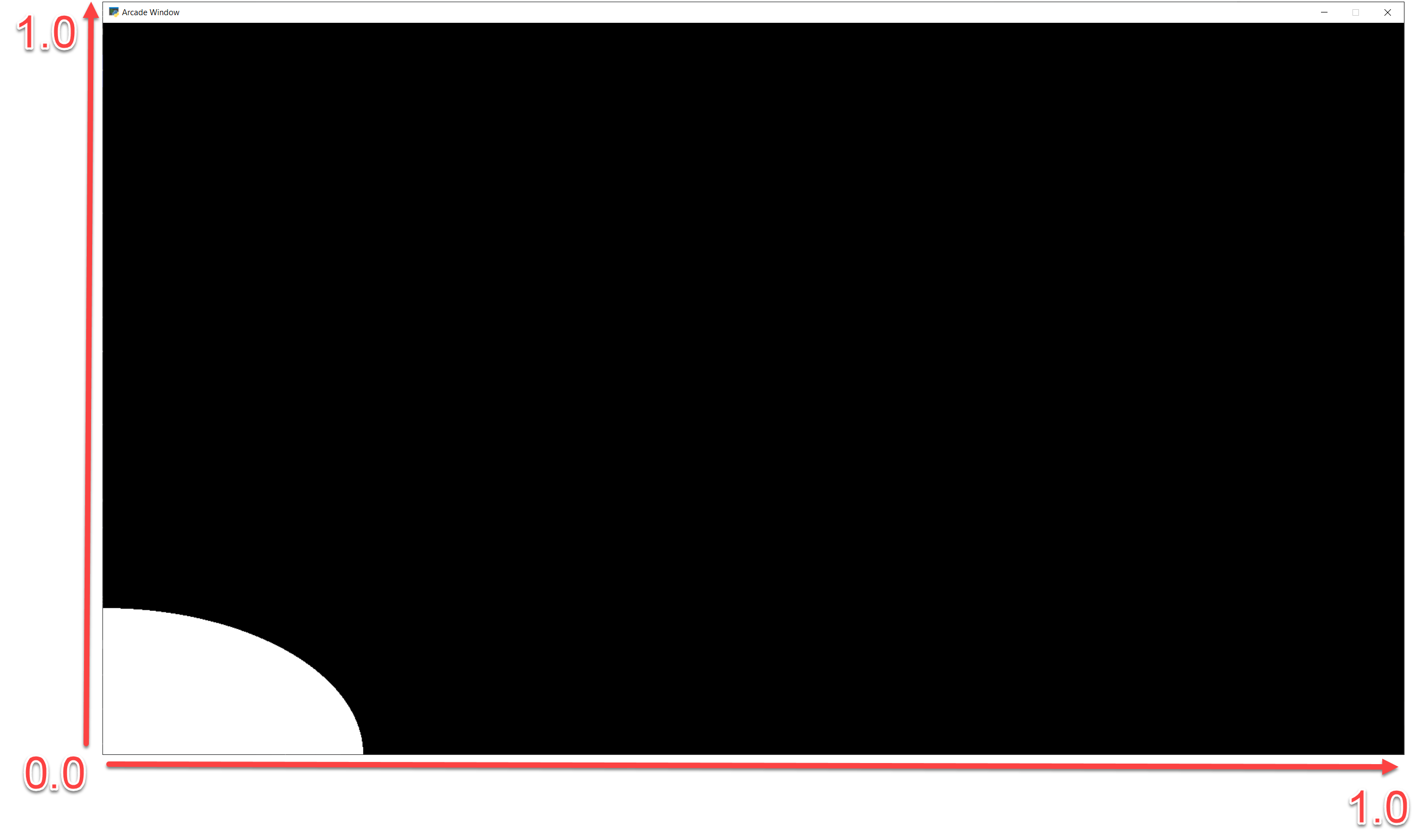Other default variables you can use:

uniform vec3 iResolution;
uniform float iTime;
uniform float iTimeDelta;
uniform float iFrame;
uniform float iChannelTime;
uniform vec4 iMouse;
uniform vec4 iDate;
uniform float iSampleRate;
uniform vec3 iChannelResolution;
uniform samplerXX iChanneli;


“Uniform” means the data is the same for each pixel the GLSL program runs on.

## Step 4: Move origin to center of screen, adjust for aspect#

Next up, we’d like to center our circle, and adjust for the aspect ratio. This will give us a (0, 0) in the middle of the screen and a perfect circle.

Center the origin#
  1 2 3 4 5 6 7 8 9 10 11 12 13 14 15 16 17 18 19 20 21 22 23 24 25 void mainImage(out vec4 fragColor, in vec2 fragCoord) { // Normalized pixel coordinates (from 0 to 1) vec2 uv = fragCoord/iResolution.xy; // Position of fragment relative to center of screen vec2 rpos = uv - 0.5; // Adjust y by aspect ratio rpos.y /= iResolution.x/iResolution.y; // How far is the current pixel from the origin (0, 0) float distance = length(rpos); // Default our color to white vec3 color = vec3(1.0, 1.0, 1.0); // Are we are 20% of the screen away from the origin? if (distance > 0.2) { // Black fragColor = vec4(0.0, 0.0, 0.0, 1.0); } else { // White fragColor = vec4(1.0, 1.0, 1.0, 1.0); } }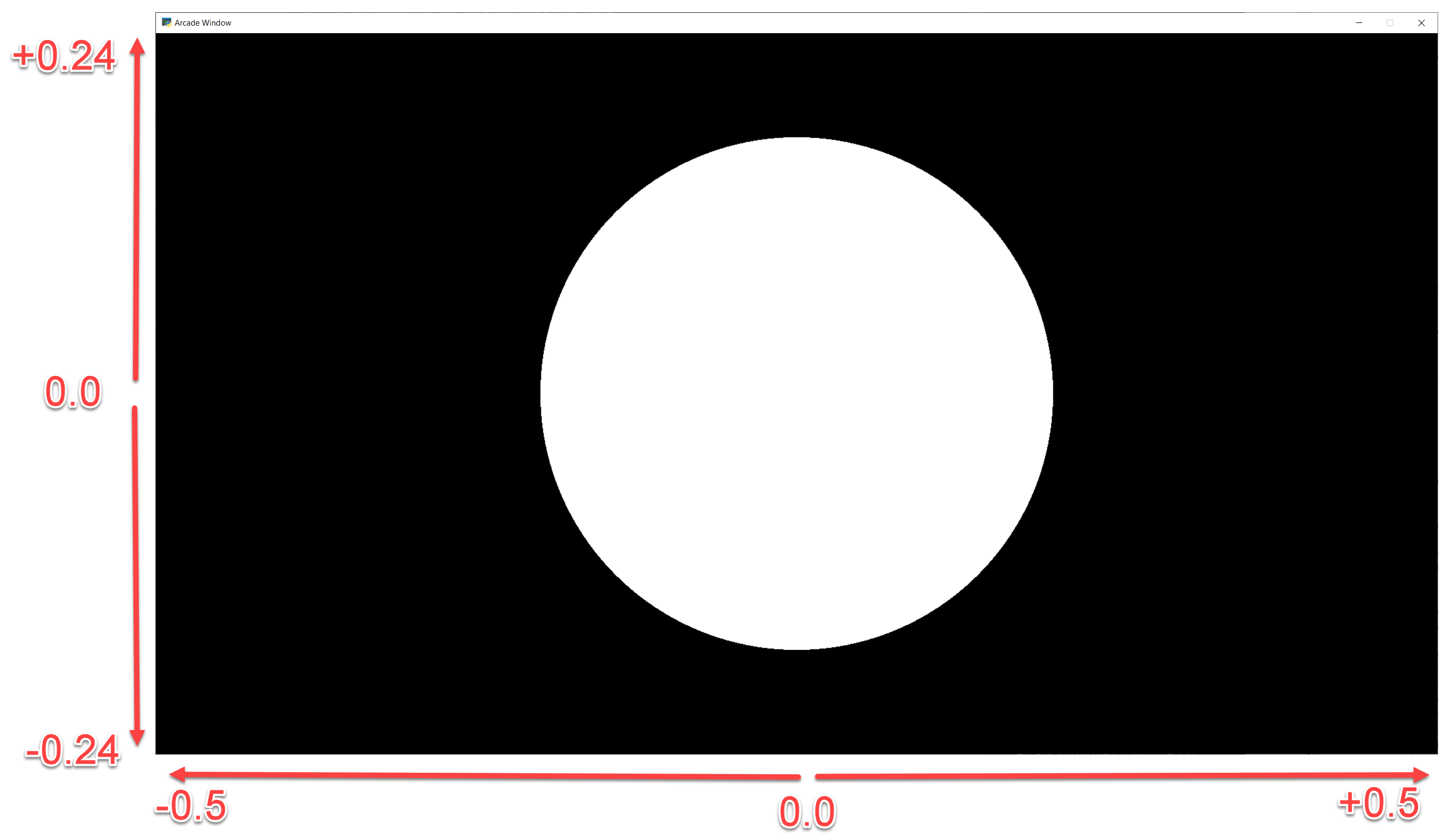We can take colors, like our white (1.0, 1.0, 1.0) and adjust their intensity by multiplying them times a float. Multiplying white times 0.5 will give us gray (0.5, 0.5, 0.5).

We can use this to create a fade effect around our circle. The inverse of the distance $$\frac{1}{d}$$ gives us a good curve. However the numbers are too large to adjust our white color. We can solve this by scaling it down. Run this, and adjust the scale value to see how it changes.

  1 2 3 4 5 6 7 8 9 10 11 12 13 14 15 16 17 18 19 20 21 22 void mainImage(out vec4 fragColor, in vec2 fragCoord) { // Normalized pixel coordinates (from 0 to 1) vec2 uv = fragCoord/iResolution.xy; // Position of fragment relative to center of screen vec2 rpos = uv - 0.5; // Adjust y by aspect ratio rpos.y /= iResolution.x/iResolution.y; // How far is the current pixel from the origin (0, 0) float distance = length(rpos); // Use an inverse 1/distance to set the fade float scale = 0.02; float strength = 1.0 / distance * scale; // Fade our white color vec3 color = strength * vec3(1.0, 1.0, 1.0); // Output to the screen fragColor = vec4(color, 1.0); }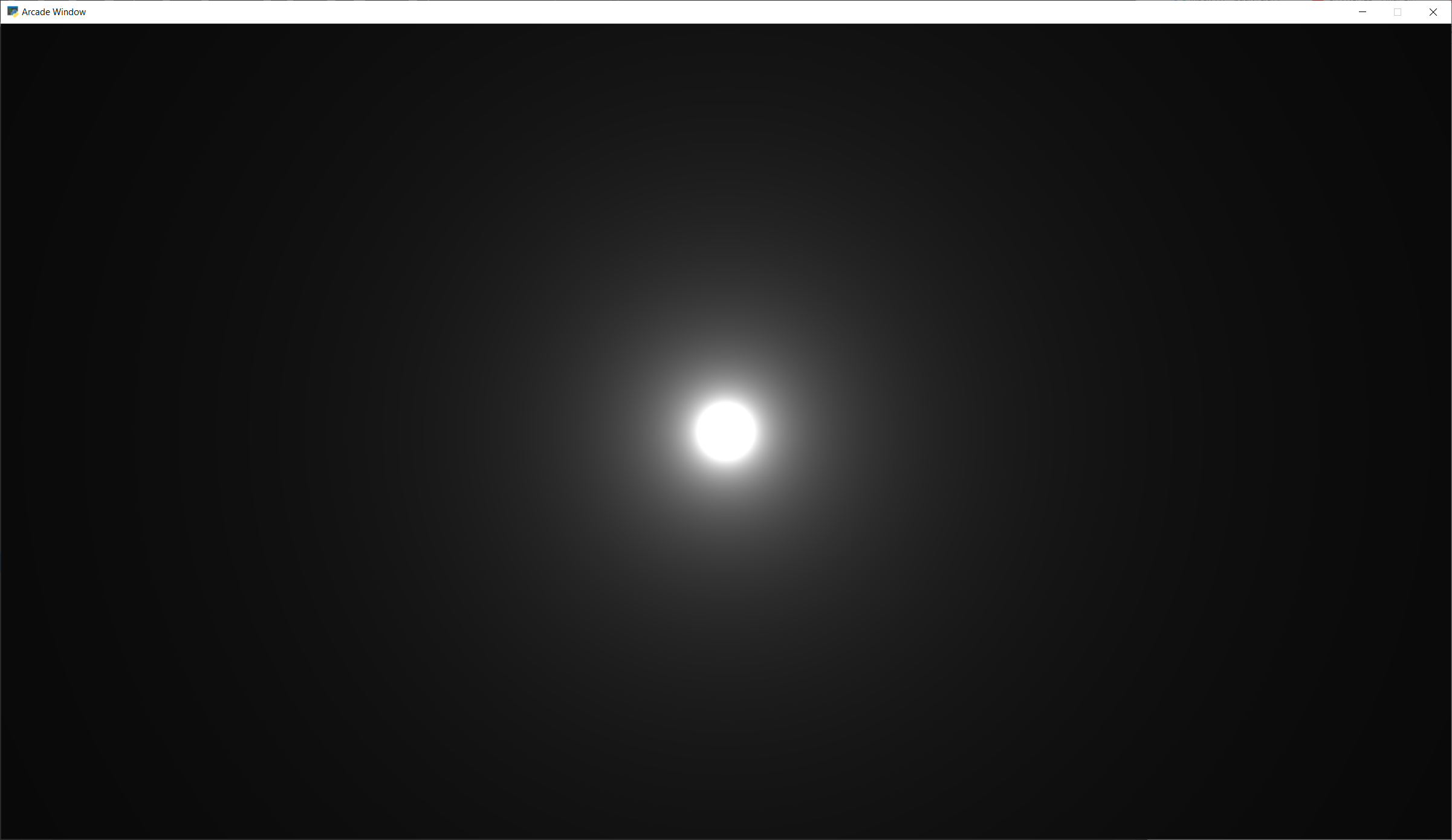We can use an exponent to adjust how steep or shallow that curve is. If we use 1.0 it will be the same, 0.5 will cause it to fade out slower, 1.5 will fade faster.

We can also change our color to orange.

  1 2 3 4 5 6 7 8 9 10 11 12 13 14 15 16 17 18 19 20 21 22 23 void mainImage(out vec4 fragColor, in vec2 fragCoord) { // Normalized pixel coordinates (from 0 to 1) vec2 uv = fragCoord/iResolution.xy; // Position of fragment relative to center of screen vec2 rpos = uv - 0.5; // Adjust y by aspect ratio rpos.y /= iResolution.x/iResolution.y; // How far is the current pixel from the origin (0, 0) float distance = length(rpos); // Use an inverse 1/distance to set the fade float scale = 0.02; float fade = 1.5; float strength = pow(1.0 / distance * scale, fade); // Fade our orange color vec3 color = strength * vec3(1.0, 0.5, 0.0); // Output to the screen fragColor = vec4(color, 1.0); }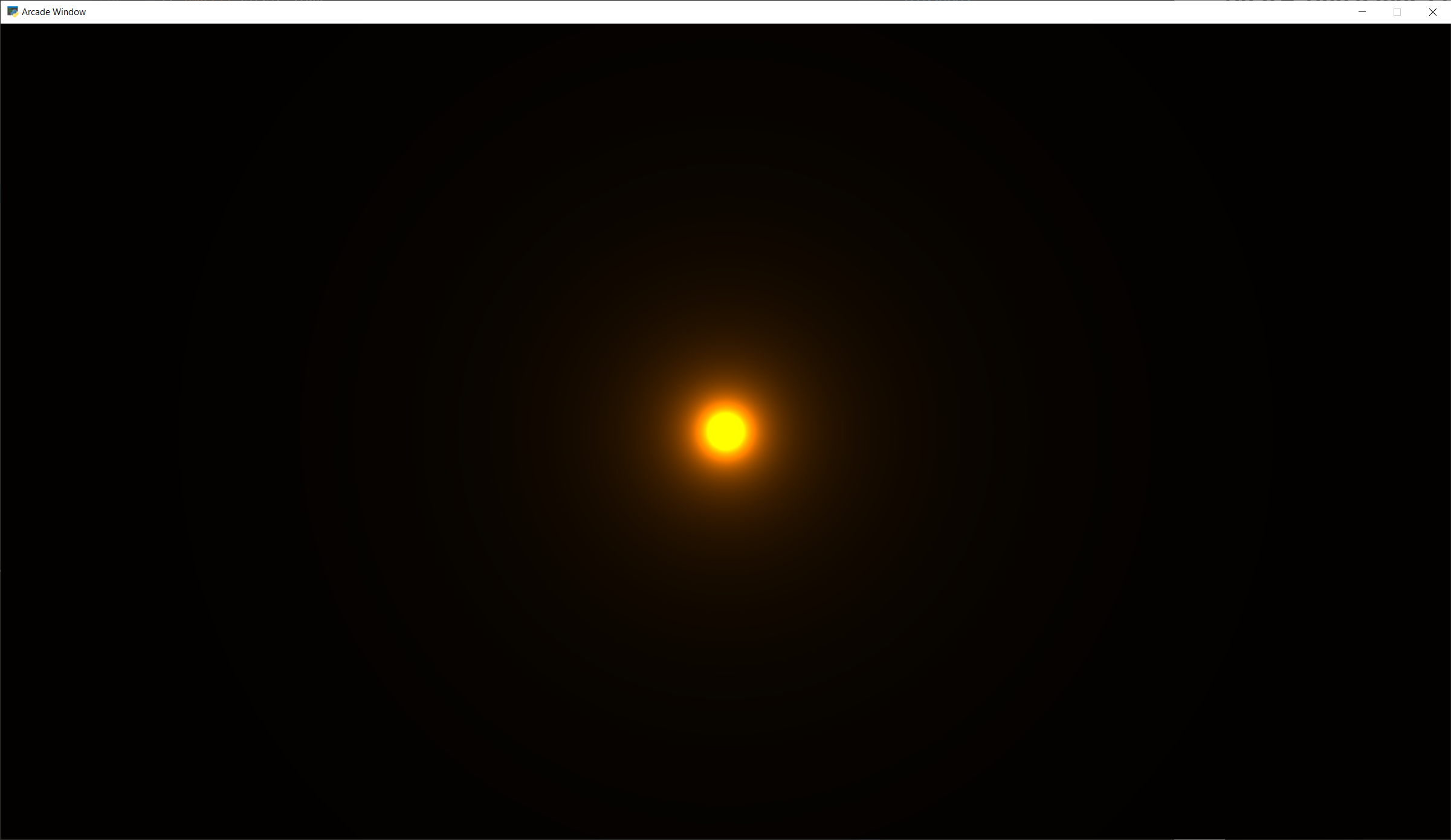## Step 7: Tone mapping#

Once we add color, the glow looks a bit off. We can do “tone mapping” with a bit of math if you like the look better.

Tone mapping#
  1 2 3 4 5 6 7 8 9 10 11 12 13 14 15 16 17 18 19 20 21 22 23 24 25 26 void mainImage(out vec4 fragColor, in vec2 fragCoord) { // Normalized pixel coordinates (from 0 to 1) vec2 uv = fragCoord/iResolution.xy; // Position of fragment relative to center of screen vec2 rpos = uv - 0.5; // Adjust y by aspect ratio rpos.y /= iResolution.x/iResolution.y; // How far is the current pixel from the origin (0, 0) float distance = length(rpos); // Use an inverse 1/distance to set the fade float scale = 0.02; float fade = 1.1; float strength = pow(1.0 / distance * scale, fade); // Fade our orange color vec3 color = strength * vec3(1.0, 0.5, 0); // Tone mapping color = 1.0 - exp( -color ); // Output to the screen fragColor = vec4(color, 1.0); }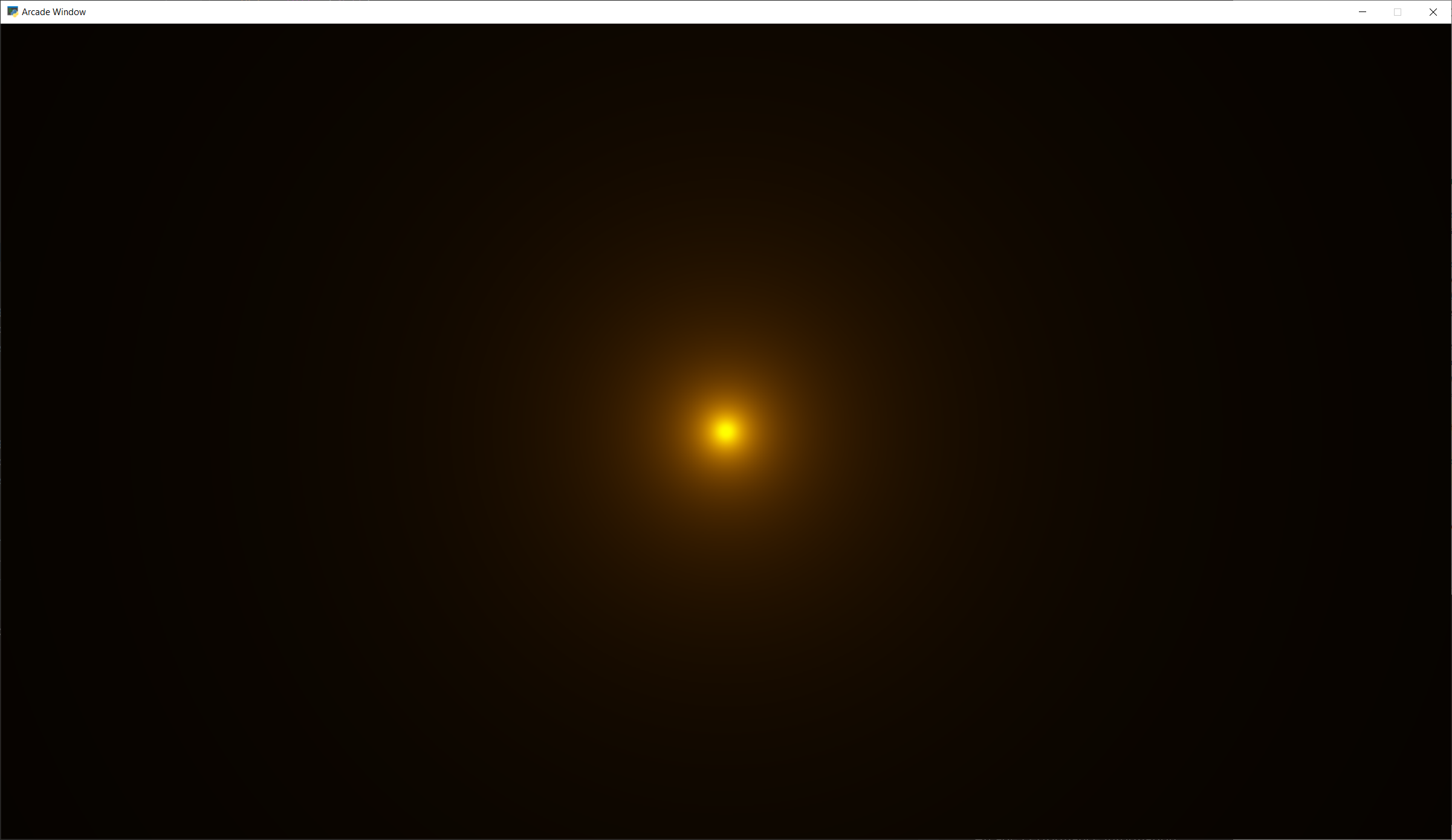## Step 8: Positioning the glow#

What if we want to position the glow at a certain spot? Send an x, y to center on? What if we want to control the color of the glow too?

We can send data to our shader using uniforms. The data we send will be the same (uniform) for each pixel rendered by the shader. The uniforms can easily be set in our Python program:

  1 2 3 4 5 6 7 8 9 10 11 12 13 14 15 16 17 18 19 20 21 22 23 24 25 import arcade from arcade.experimental import Shadertoy # Derive an application window from Arcade's parent Window class class MyGame(arcade.Window): def __init__(self): # Call the parent constructor super().__init__(width=1920, height=1080) # Load a file and create a shader from it shader_file_path = "circle_6.glsl" window_size = self.get_size() self.shadertoy = Shadertoy.create_from_file(window_size, shader_file_path) def on_draw(self): # Set uniform data to send to the GLSL shader self.shadertoy.program['pos'] = self.mouse["x"], self.mouse["y"] self.shadertoy.program['color'] = arcade.get_three_float_color(arcade.color.LIGHT_BLUE) # Run the GLSL code self.shadertoy.render() if __name__ == "__main__": MyGame() arcade.run() 

Then we can use those uniforms in our shader:

Glow follows mouse, and color can be changed.#
  1 2 3 4 5 6 7 8 9 10 11 12 13 14 15 16 17 18 19 20 21 22 23 24 25 26 27 28 29 30 uniform vec2 pos; uniform vec3 color; void mainImage(out vec4 fragColor, in vec2 fragCoord) { // Normalized pixel coordinates (from 0 to 1) vec2 uv = fragCoord/iResolution.xy; vec2 npos = pos/iResolution.xy; // Position of fragment relative to specified position vec2 rpos = npos - uv; // Adjust y by aspect ratio rpos.y /= iResolution.x/iResolution.y; // How far is the current pixel from the origin (0, 0) float distance = length(rpos); // Use an inverse 1/distance to set the fade float scale = 0.02; float fade = 1.1; float strength = pow(1.0 / distance * scale, fade); // Fade our orange color vec3 color = strength * color; // Tone mapping color = 1.0 - exp( -color ); // Output to the screen fragColor = vec4(color, 1.0); }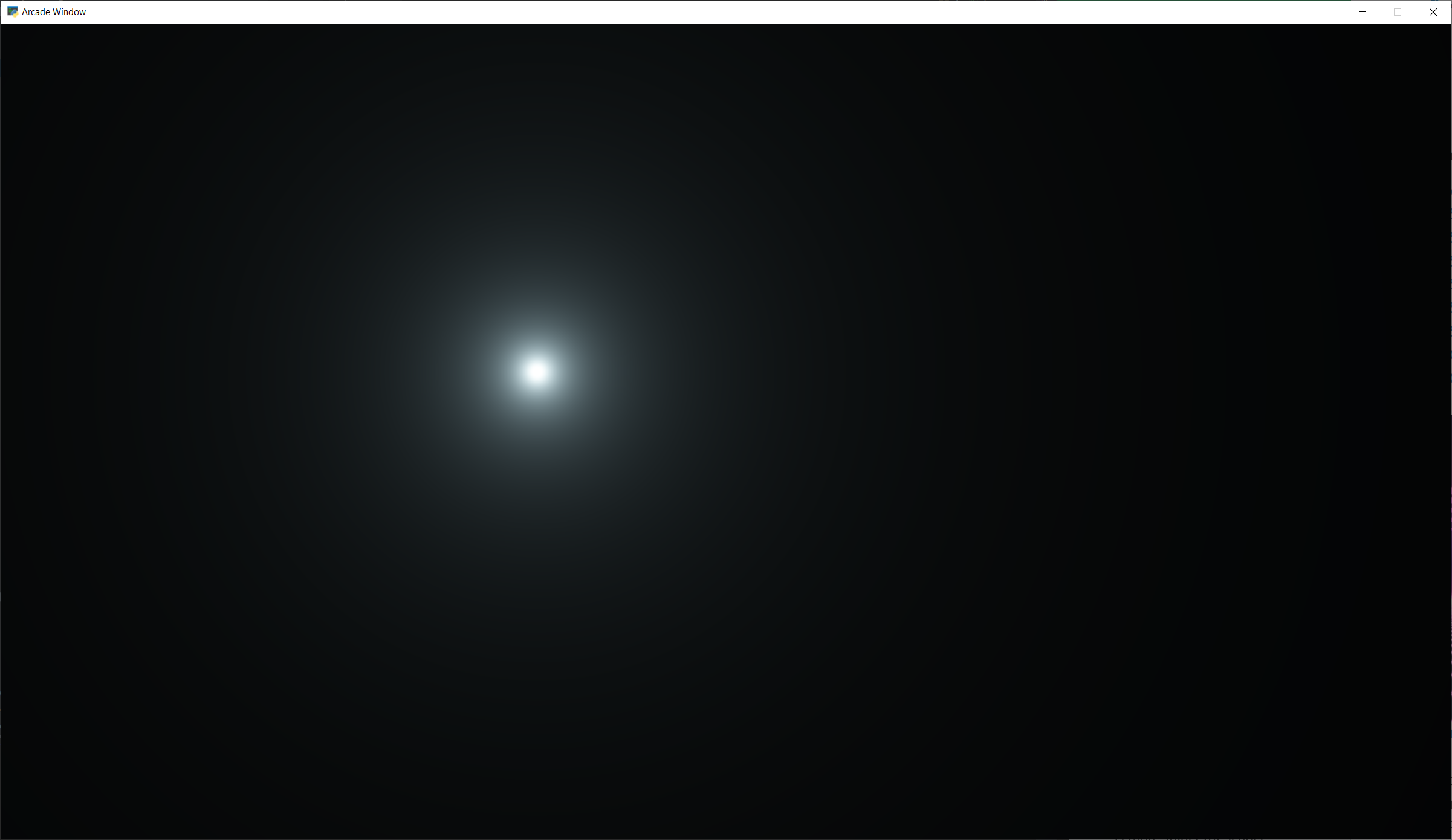Note

Built-in Uniforms

Shadertoy assumes some built-in values. These can be set during the Shadertoy.render() call. In this example I’m not using those variables because I want to show how to send any value, not just built-in ones. The built-in values:

Python Variable

GLSL Variable

time

iTime

time_delta

iTimeDelta

mouse_position

iMouse

size

frame

iFrame

An example of how they are set:

my_shader.render(time=self.time, mouse_position=mouse_position)


When resizing a window, make sure to always resize the shader as well.

## Other examples#

Here’s another Python program that loads a GLSL file and displays it:

  1 2 3 4 5 6 7 8 9 10 11 12 13 14 15 16 17 18 19 20 21 22 23 24 25 26 27 28 29 30 31 32 33 34 35 36 37 import arcade from arcade.experimental import Shadertoy class MyGame(arcade.Window): def __init__(self): # Call the parent constructor super().__init__(width=1920, height=1080, title="Shader Demo", resizable=True) # Keep track of total run-time self.time = 0.0 # File name of GLSL code # file_name = "fractal_pyramid.glsl" # file_name = "cyber_fuji_2020.glsl" file_name = "earth_planet_sky.glsl" # file_name = "flame.glsl" # file_name = "star_nest.glsl" # Create a shader from it self.shadertoy = Shadertoy(size=self.get_size(), main_source=open(file_name).read()) def on_draw(self): self.clear() mouse_pos = self.mouse["x"], self.mouse["y"] self.shadertoy.render(time=self.time, mouse_position=mouse_pos) def on_update(self, dt): # Keep track of elapsed time self.time += dt if __name__ == "__main__": MyGame() arcade.run()